Select Page

# MCQ Answers for CBSE 12 Science Maths Vector Algebra in English

MCQ Answers for CBSE 12 Science Maths Vector Algebra in English to enable students to get Answers in a narrative video format for the specific question.

Expert Teacher provides MCQ Answers for CBSE 12 Science Maths Vector Algebra through Video Answers in English language. This video solution will be useful for students to understand how to write an answer in exam in order to score more marks. This teacher uses a narrative style for a question from Vector Algebra not only to explain the proper method of answering question, but deriving right answer too.

Please find the question below and view the Answer in a narrative video format.

Question:

## Similar Questions from CBSE, 12th Science, Maths, Vector Algebra

Question 1 :  Write the value of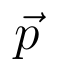for which the vectorsandare parallel vectors.  (View Answer Video)

Question 2 :  If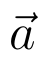and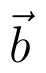denote the position vectors of points A and B respectively and C is a point on AB such that AC = 2 CB, then write the position vector of C.  (View Answer Video)

Question 3 :  Write a vector in the direction of the vectorthat has magnitude 9 units.   (View Answer Video)

Question 4 : Find the value of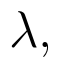if the points with position vectorsandare coplanar.  (View Answer Video)

Question 5 : Find |x|, if for a unit vector a,(x - a).(x + a) = 12. (View Answer Video)

### Determinant

Question 1 :  If A is a square matrix of order 3 and |A| = 5 then |3A| = ? (View Answer Video)

Question 3 : The following system of equations has x + 3y + 3z = 2, x + 4y + 3z = 1, x + 3y + 4z = 2,

Question 4 : Evaluate the determinant:. (View Answer Video)

Question 5 :  Using the properties of determinants, evaluate. (View Answer Video)

### Integrals

Question 1 : Evaluate :(View Answer Video)

Question 2 : Evaluate :(View Answer Video)

Question 3 : Find the integral of the function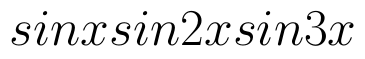. (View Answer Video)

Question 4 : Find :(View Answer Video)

Question 5 : Find the integral of the function. (View Answer Video)

### Matrices

Question 1 : If, then find "a". (View Answer Video)

Question 2 : Find the value of X, ifand. (View Answer Video)

Question 3 : Compute:. (View Answer Video)

Question 4 : Given,, find the value of y. (View Answer Video)

Question 5 : Find the value of y,  from the equation:. (View Answer Video)